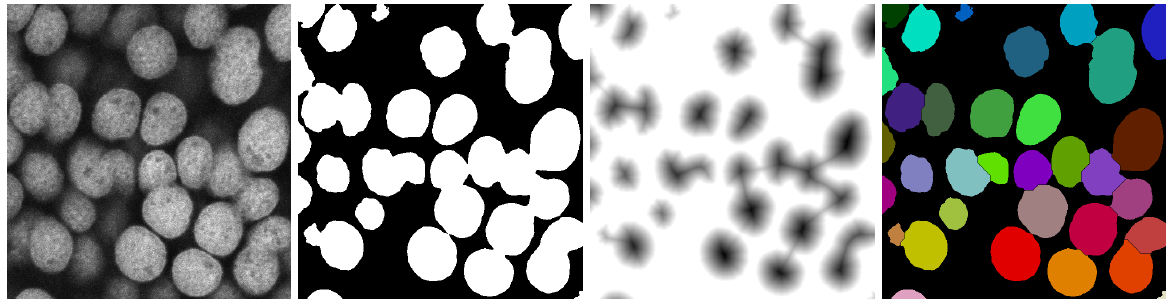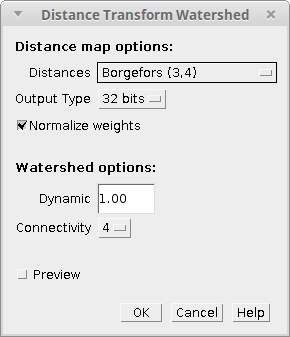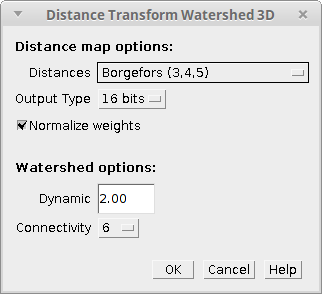# Distance Transform WatershedThe content of this page has not been vetted since shifting away from MediaWiki. If you’d like to help, check out the how to help guide!Software IJPB-plugins Source on GitHub

## Introduction

A classic way of separating touching objects in binary images makes use of the distance transform and the watershed method. The idea is to create a border as far as possible from the center of the overlapping objects. This strategy works very well on rounded objects and it is called Distance Transform Watershed. It consists on calculating the distance transform of the binary image, inverting it (so the darkest parts of the image are the centers of the objects) and then applying watershed on it using the original image as mask (see figure below). In our implementation, we include an option to use watershed with extended minima so the user can control the number of object splits and merges.Basics of the Distance Transform Watershed algorithm. From left to right: sample image of touching DAPI stained cell nuclei from a confocal laser scanning microscope, binary mask calculated after filtering and thresholding input image, inverse of the distance transform applied to the binary mask (Chamfer distance map using normalized Chessknight weights and 32-bit output) and resulting labeled image after applying watershed to the inverse distance image using the binary mask (dynamic of 1 and 4-connectivity).

MorphoLibJ provides two plugins under the PluginsMorphoLibJBinary Images… menu to apply this strategy on 2D and 3D images:

## Distance Transform Watershed

Distance Transform Watershed needs one 2D 8-bit binary image to run. If that’s the case, a dialog like the following will pop up:The plugin parameters are divided between the distance transform and the watershed options:

• Distance map options:
• Distances: allows selecting among a pre-defined set of weights that can be used to compute the distance transform using Chamfer approximations of the Euclidean metric. They affect the location but specially the shape of the border in the final result. The options are:
• Chessboard (1,1): weight equal to 1 for all neighbors.
• City-Block (1,2): weights 1 for orthogonal neighbors and 2 for diagonal neighbors.
• Quasi-Euclidean (1,1.41): weights 1 for orthogonal neighbors and $$\sqrt{2}$$ for diagonal neighbors.
• Borgefors (3,4): weights 3 for orthogonal neighbors and 4 for diagonal neighbors (best approximation of Euclidean distance for 3-by-3 masks).
• Weights (2,3): weights 2 for orthogonal neighbors and 3 for diagonal neighbors.
• Weights (5,7): weights 5 for orthogonal neighbors and 7 for diagonal neighbors.
• Chessknight (5,7,11): weights 5 for orthogonal neighbors and 7 for diagonal neighbors, and 11 for chess-knight moves (best approximation for 5-by-5 masks).
• Output type: 16 or 32-bit, to calculate distance with short or float precision.
• Normalize weights: indicates whether the resulting distance map should be normalized (divide distances by the first Chamfer weight).
• Watershed options:
• Dynamic: same as in the Morphological Segmentation plugin, this is the dynamic of intensity for the search of regional minima in the inverse of the distance transform image. Basically, by increasing its value there will be more object merges and by decreasing it there will be more object splits.
• Connectivity: pixel connectivity (4 or 8). Selecting non-diagonal connectivity (4) usually provides more rounded objects.

Finally, the result with the current plugin configuration can be visualize clicking on the Preview option.

Result: 2D 32-bit label image (one index value per object).

## Distance Transform Watershed (3D)

Distance Transform Watershed 3D needs one 3D 8-bit binary image to run. If that’s the case, a dialog like the following will pop up:The parameters are the same as in the 2D version but some of them are adapted for 3D images:

• Distance map options:
• Distances: Now the available options are:
• Chessboard (1,1,1): weight equal to 1 for all neighbors.
• City-Block (1,2,3): weights 1 for orthogonal neighbors, 2 for diagonal neighbors and 3 for cube-diagonals.
• Quasi-Euclidean (1,1.41,1.73): weights 1 for orthogonal neighbors, $$\sqrt{2}$$ for diagonal neighbors and $$\sqrt{3}$$ for cube-diagonals.
• Borgefors (3,4,5): weights 3 for orthogonal neighbors, 4 for diagonal neighbors and 5 for cube-diagonals (best approximation of Euclidean distance for 3-by-3-by-3 masks).
• Output type: 16 or 32-bit, to calculate distance with short or float precision.
• Normalize weights: indicates whether the resulting distance map should be normalized (divide distances by the first Chamfer weight).
• Watershed options:
• Dynamic: same as in the 2D version, this is the dynamic of intensity for the search of regional minima in the inverse of the distance transform image. Basically, by increasing its value there will be more object merges and by decreasing it there will be more object splits.
• Connectivity: voxel connectivity (6 or 26). Selecting non-diagonal connectivity (6) usually provides more rounded objects.

As it is usual in ImageJ, no preview is provided here since we are dealing with 3D images.

Result: 3D 32-bit label image (one index value per object).

## Installation

The Distance Transform Watershed plugins (2D and 3D) are part of the MorphoLibJ library. To install them, you just need to add the IJPB-plugins update site:

1) Select HelpUpdate… from the Fiji menu to start the updater.

2) Click on Manage update sites. This brings up a dialog where you can activate additional update sites.

3) Activate the IJPB-plugins update site and close the dialog. Now you should see an additional jar file for download.

4) Click Apply changes and restart Fiji.

You should now find the plugin under the sub-menu PluginsMorphoLibJSegmentation.

Note: the Distance Transform Watershed plugins are only two of the plugins included in the MorphoLibJ suite. By following these installation steps, you will be installing as well the rest of plugins in the suite.

1. David Legland, Ignacio Arganda-Carreras, Philippe Andrey; MorphoLibJ: integrated library and plugins for mathematical morphology with ImageJ. Bioinformatics 2016; 32 (22): 3532-3534. doi: 10.1093/bioinformatics/btw413